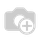# How to use the value of a selection field instead of its key in a python function ?

Hi !

I have a fields.selection, and I want to use the value of this field in a python function.

But, when I pass the field to this function, I get a string, the key...

So, how can I get the value instead ?

Thanks !

EDIT

Hi ! New week, already no answer for this... I wonder if a way to do this really exists...• model is the name of the model, example: 'account.invoice'
• field is the name of the field inside the model, example: 'state'
• field_val is the value of that field, example: 'draft'

Code:

``````dict(self.pool.get(model).fields_get(cr, uid, allfields=[field], context=context)[field]['selection'])[field_val]
``````

Example:

``````dict(self.pool.get('account.invoice').fields_get(cr, uid, allfields=['state'], context=context)['state']['selection'])['draft']
``````Hum, this is quite unreadable, can you give me an example ?

test=dict(self.pool.get('sale.order').fields_get(cr, uid, allfields=['state'], context=context))['state']['selection']

``````    print "***************order",test                 ///////////////[('draft', u'Devis brouillon'), ('sent', u'Devis envoy\xe9'), ('cancel', u'Annul\xe9e'), ('waiting_date', u'Attente de planification'), ('progress', u'Bon de commande'), ('manual', u'Commande \xe0 facturer'), ('shipping_except', u"Incident d'exp\xe9dition"), ('invoice_except', u'Incident de facturation'), ('done', u'Termin\xe9e')]
``````

I use another technique.

First I define the list of pairs code/value

`VALUES = [('a', 'A'), ('b','B'), ('c','C')]`

I use it into my field definition

`f = fields.Selection(VALUES)`

and to return value, given the code, with a dict

`dict(VALUES)[self.f]`

or with a simple loop

`for item in VALUES:    if item == self.f:         return item`
1 CommentThe V8 way:

use the @api.one decorator

• current model :value = dict(self.fields_get(allfields=['state'])['state']['selection'])['key']

• other model : value = dict(self.env['your.model'].fields_get(allfields=['state'])['state']['selection'])['key']

Hope this help somebody..you can use this.

dict(self._fields['your_selection_field'].selection).get(self.your_selection_field)I use another technique.

First I define the list of pairs code/value

`VALUES = [('a', 'A'), ('b','B'), ('c','C')]`

I use it into my field definition

`f = fields.Selection(VALUES)`

and to return value, given the code, with a dict

`dict(VALUES)[self.f]`

or with a simple loop

`for item in VALUES:    if item == self.f:`
1 Commentthere must be some error: i can't explain myself this duplicate answer...